# College Algebra Sixth Edition James Stewart Lothar Redlin

• Slides: 48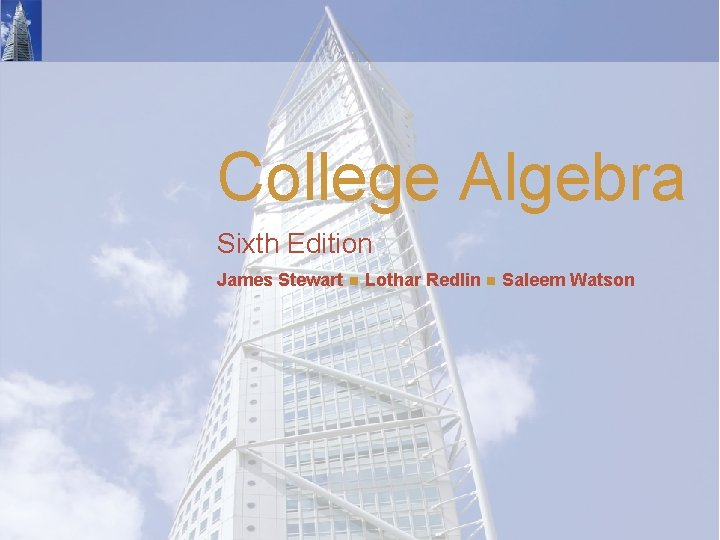College Algebra Sixth Edition James Stewart Lothar Redlin Saleem Watson8 Sequences and Series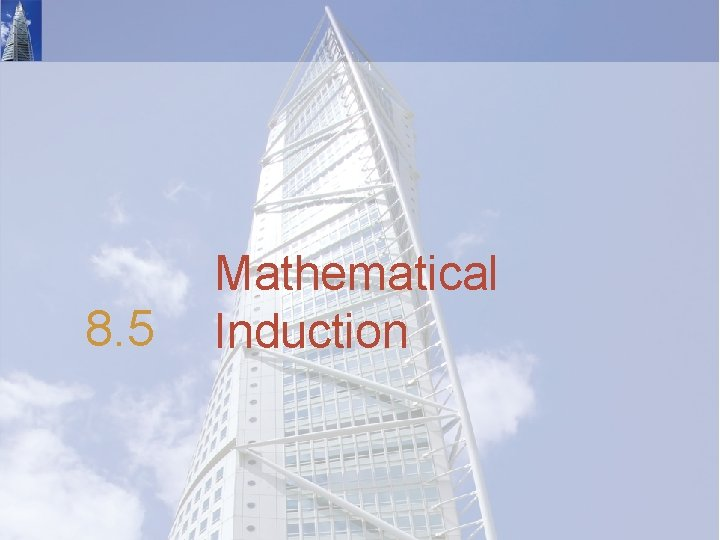8. 5 Mathematical Induction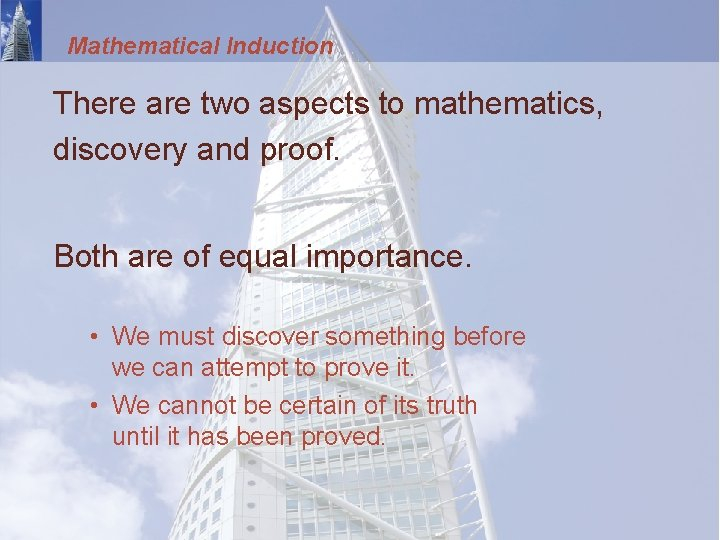Mathematical Induction There are two aspects to mathematics, discovery and proof. Both are of equal importance. • We must discover something before we can attempt to prove it. • We cannot be certain of its truth until it has been proved.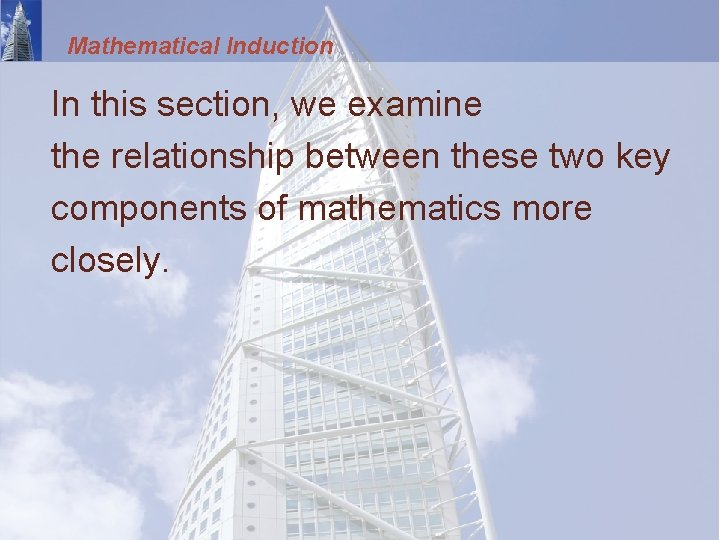Mathematical Induction In this section, we examine the relationship between these two key components of mathematics more closely.Conjecture and Proof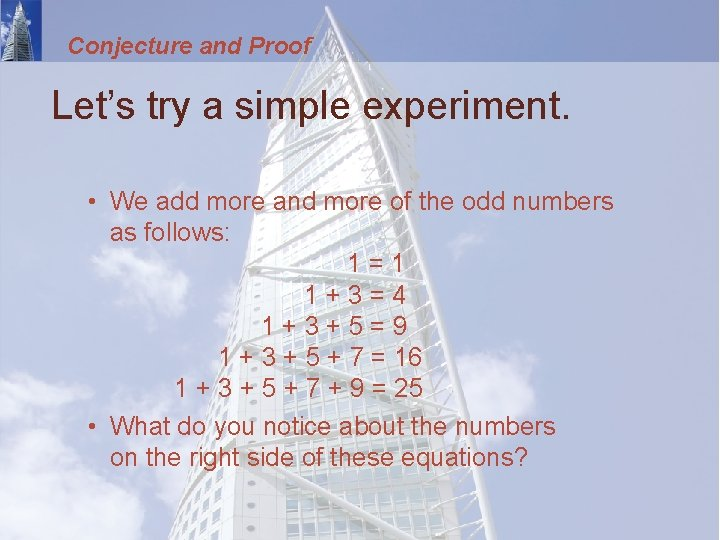Conjecture and Proof Let’s try a simple experiment. • We add more and more of the odd numbers as follows: 1=1 1+3=4 1+3+5=9 1 + 3 + 5 + 7 = 16 1 + 3 + 5 + 7 + 9 = 25 • What do you notice about the numbers on the right side of these equations?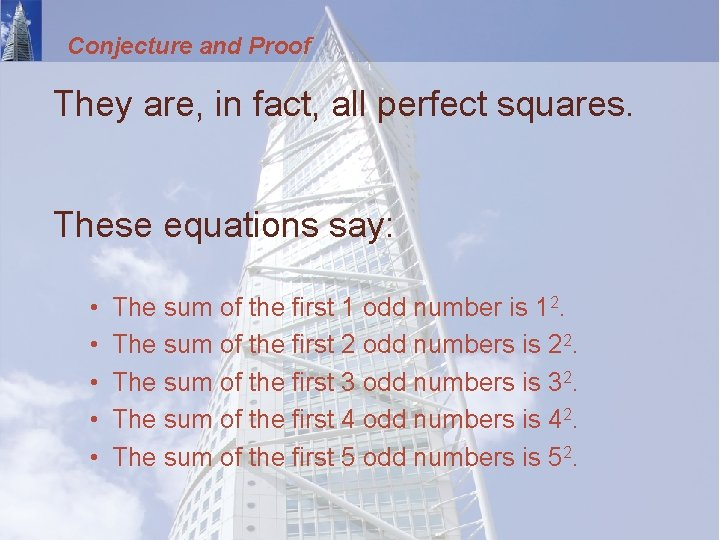Conjecture and Proof They are, in fact, all perfect squares. These equations say: • • • The sum of the first 1 odd number is 12. The sum of the first 2 odd numbers is 22. The sum of the first 3 odd numbers is 32. The sum of the first 4 odd numbers is 42. The sum of the first 5 odd numbers is 52.Conjecture and Proof This leads naturally to the following question: Is it true that, for every natural number n, the sum of the first n odd numbers is n 2? • Could this remarkable property be true?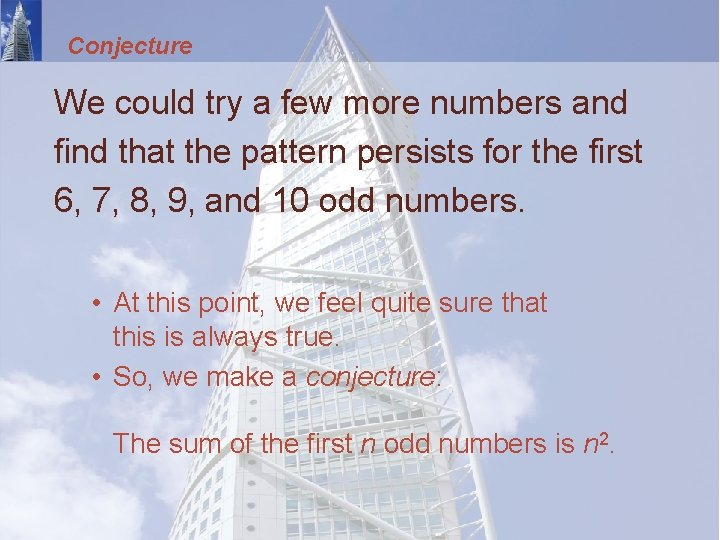Conjecture We could try a few more numbers and find that the pattern persists for the first 6, 7, 8, 9, and 10 odd numbers. • At this point, we feel quite sure that this is always true. • So, we make a conjecture: The sum of the first n odd numbers is n 2.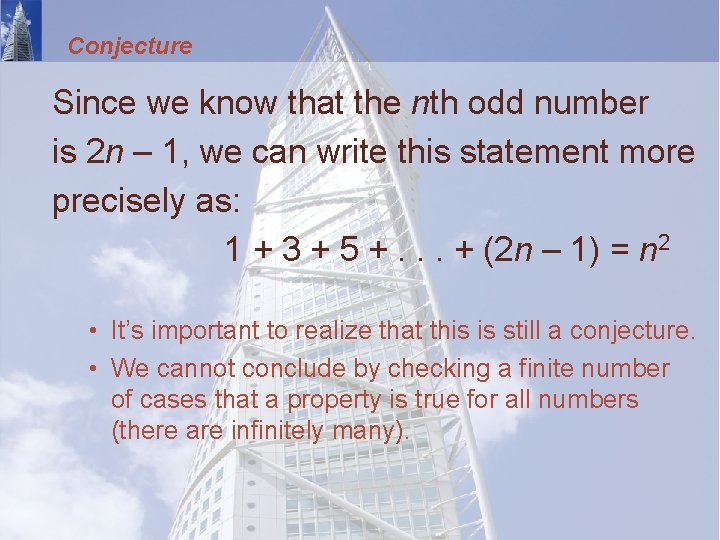Conjecture Since we know that the nth odd number is 2 n – 1, we can write this statement more precisely as: 1 + 3 + 5 +. . . + (2 n – 1) = n 2 • It’s important to realize that this is still a conjecture. • We cannot conclude by checking a finite number of cases that a property is true for all numbers (there are infinitely many).Conjecture Let’s see this more clearly. Suppose someone tells us he has added up the first trillion odd numbers and found that they do not add up to 1 trillion squared. • What would you tell this person?Conjecture It would be silly to say that you’re sure it’s true because you’ve already checked the first five cases. • You could, however, take out paper and pencil and start checking it yourself. (This would probably take the rest of your life. )Conjecture The tragedy would be that, after completing this task, you would still not be sure of the truth of the conjecture! • Do you see why?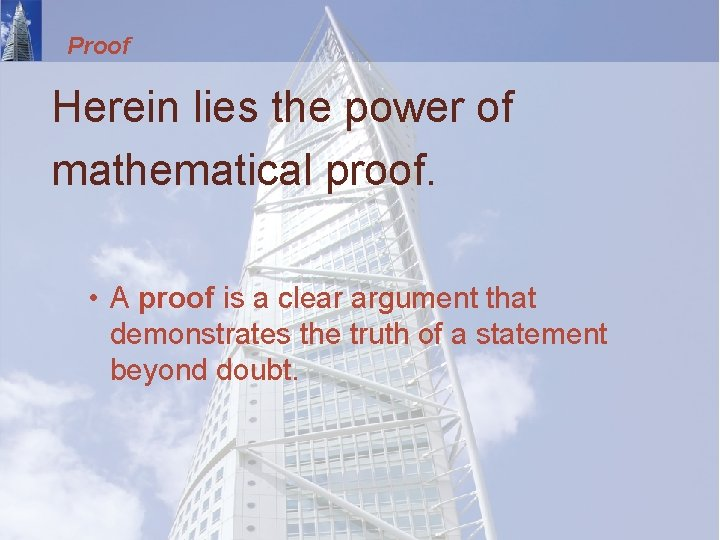Proof Herein lies the power of mathematical proof. • A proof is a clear argument that demonstrates the truth of a statement beyond doubt.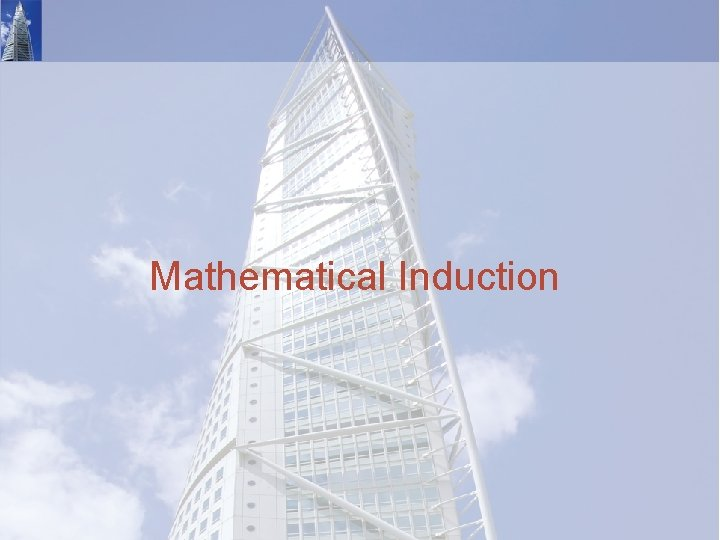Mathematical Induction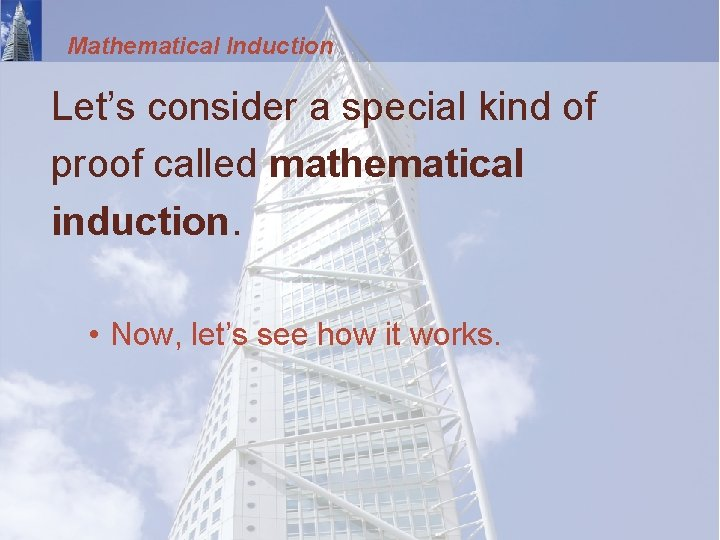Mathematical Induction Let’s consider a special kind of proof called mathematical induction. • Now, let’s see how it works.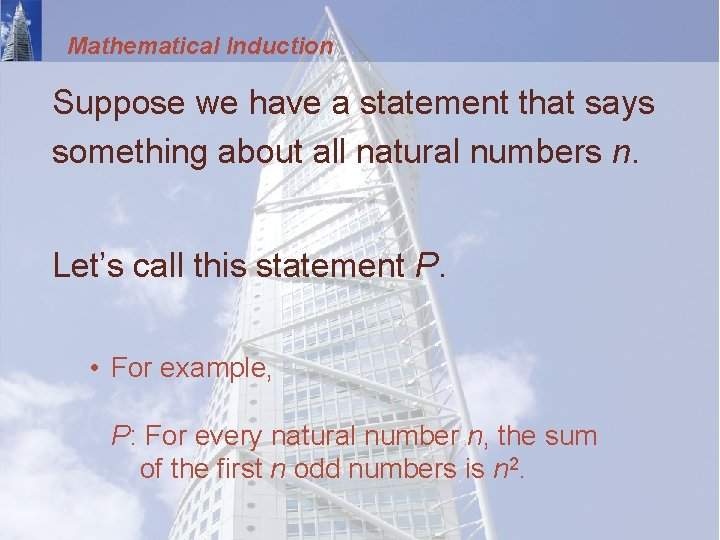Mathematical Induction Suppose we have a statement that says something about all natural numbers n. Let’s call this statement P. • For example, P: For every natural number n, the sum of the first n odd numbers is n 2.Mathematical Induction Since this statement is about all natural numbers, it contains infinitely many statements. • We will call them P(1), P(2), . . P(1): The sum of the first 1 odd number is 12. P(2): The sum of the first 2 odd numbers is 22. P(3): The sum of the first 3 odd numbers is 32. . . .Mathematical Induction How can we prove all of these statements at once? • Mathematical induction is a clever way of doing so.Mathematical Induction The crux of the idea is: • Suppose we can prove that, whenever one of these statements is true, then the one following it in the list is also true. • That is, For every k, if P(k) is true, then P(k + 1) is true. • This is called the induction step since it leads us from the truth of one statement to the next.Mathematical Induction Now, suppose that we can also prove that P(1) is true. • The induction step now leads us through the following chain of statements. • P(1) is true, so P(2) is true, so P(3) is true, so P(4) is true. . . .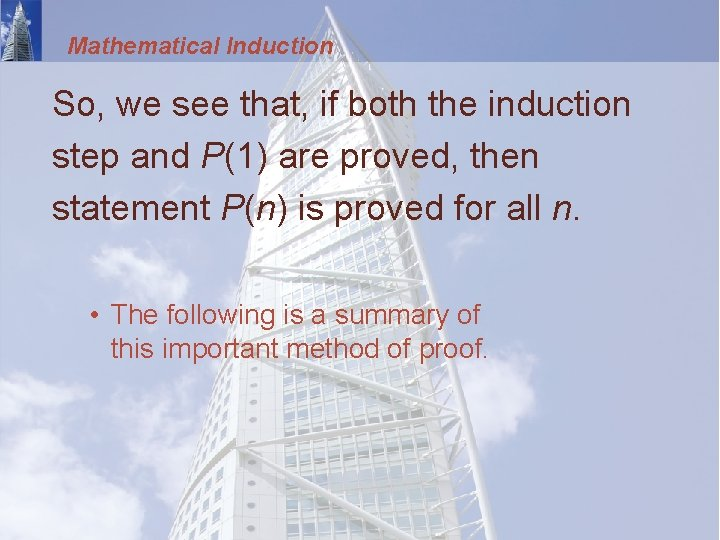Mathematical Induction So, we see that, if both the induction step and P(1) are proved, then statement P(n) is proved for all n. • The following is a summary of this important method of proof.Principle of Mathematical Induction For each natural number n, let P(n) be a statement depending on n. • Suppose that following two conditions are satisfied. 1. P(1) is true. 2. For every natural number k, if P(k) is true, then P(k + 1) is true. • Then, P(n) is true for all natural numbers n.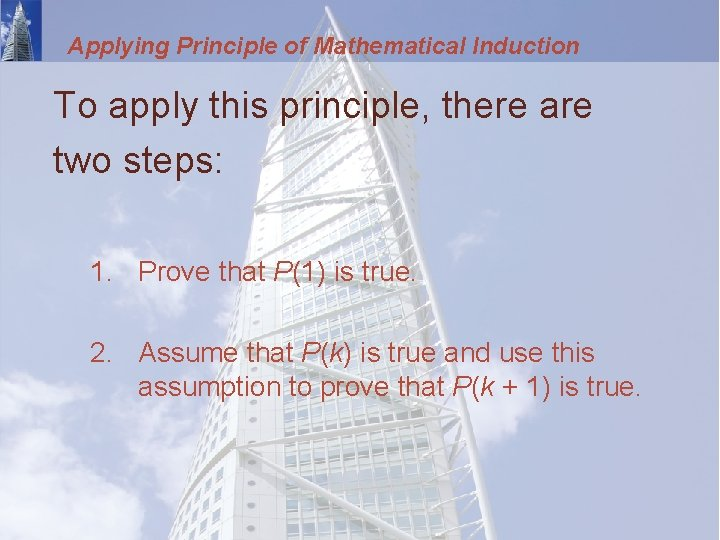Applying Principle of Mathematical Induction To apply this principle, there are two steps: 1. Prove that P(1) is true. 2. Assume that P(k) is true and use this assumption to prove that P(k + 1) is true.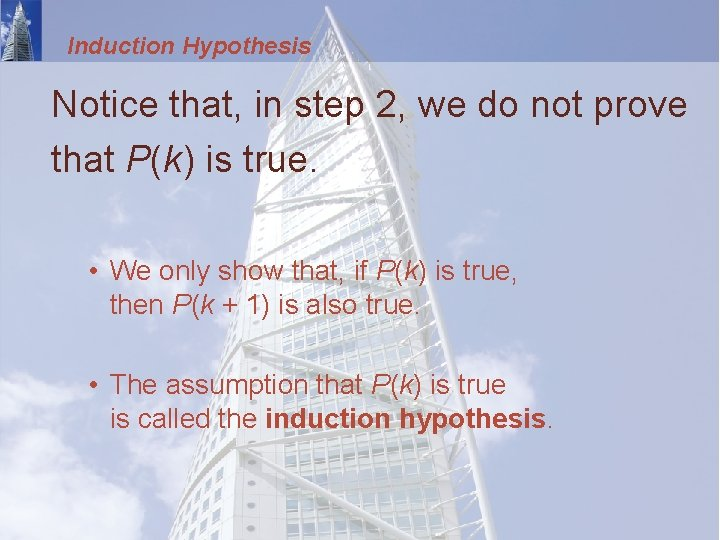Induction Hypothesis Notice that, in step 2, we do not prove that P(k) is true. • We only show that, if P(k) is true, then P(k + 1) is also true. • The assumption that P(k) is true is called the induction hypothesis.Mathematical Induction We now use mathematical induction to prove that the conjecture we made at the beginning of this section is true.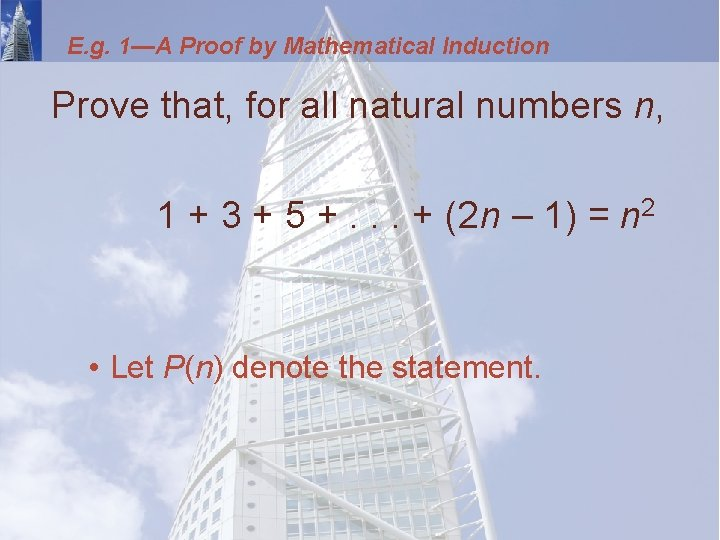E. g. 1—A Proof by Mathematical Induction Prove that, for all natural numbers n, 1 + 3 + 5 +. . . + (2 n – 1) = n 2 • Let P(n) denote the statement.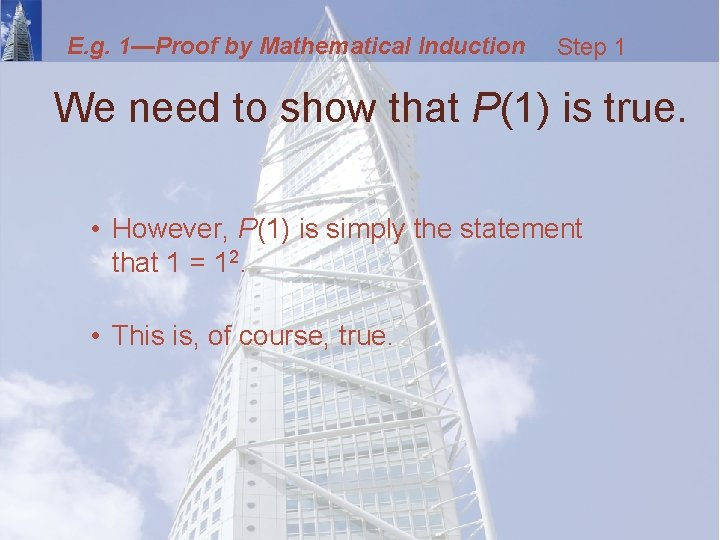E. g. 1—Proof by Mathematical Induction Step 1 We need to show that P(1) is true. • However, P(1) is simply the statement that 1 = 12. • This is, of course, true.E. g. 1—Proof by Mathematical Induction Step 2 We assume that P(k) is true. • Thus, our induction hypothesis is: 1 + 3 + 5 +. . . + (2 k – 1) = k 2E. g. 1—Proof by Mathematical Induction Step 2 We want to use this to show that P(k +1) is true. • That is, 1 + 3 + 5 +. . . + (2 k – 1) + [2(k + 1) – 1] = (k + 1)2 • Note that we get P(k + 1) by substituting k + 1 for each n in the statement P(n).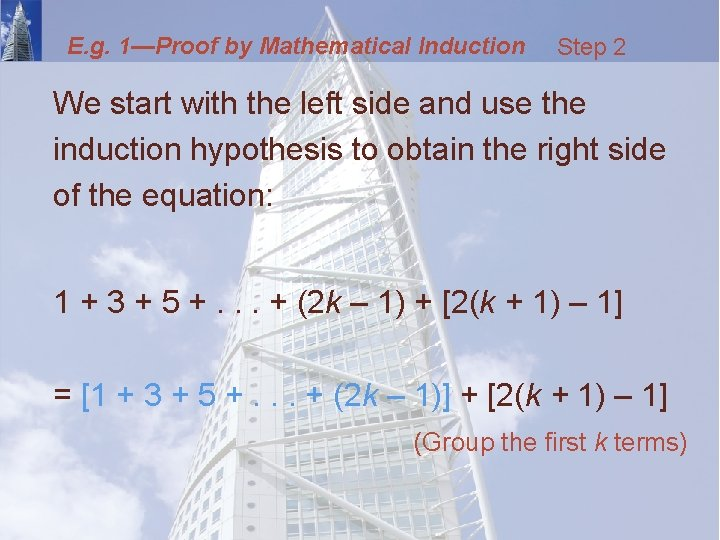E. g. 1—Proof by Mathematical Induction Step 2 We start with the left side and use the induction hypothesis to obtain the right side of the equation: 1 + 3 + 5 +. . . + (2 k – 1) + [2(k + 1) – 1] = [1 + 3 + 5 +. . . + (2 k – 1)] + [2(k + 1) – 1] (Group the first k terms)E. g. 1—Proof by Mathematical Induction Step 2 = k 2 + [2(k + 1) – 1] Induction Hypothesis = k 2 + [2 k + 2 – 1] Distributive property = k 2 + 2 k + 1 Simplify = (k + 1)2 Factor • Thus, P(k + 1) follows from P(k). • This completes the induction step.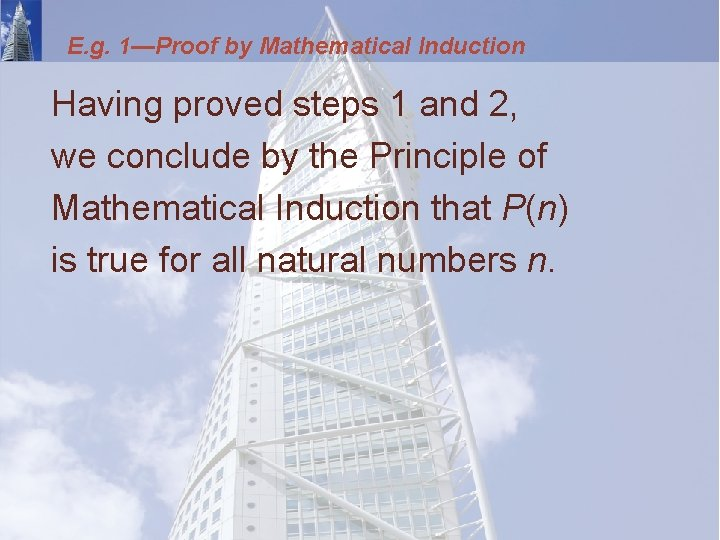E. g. 1—Proof by Mathematical Induction Having proved steps 1 and 2, we conclude by the Principle of Mathematical Induction that P(n) is true for all natural numbers n.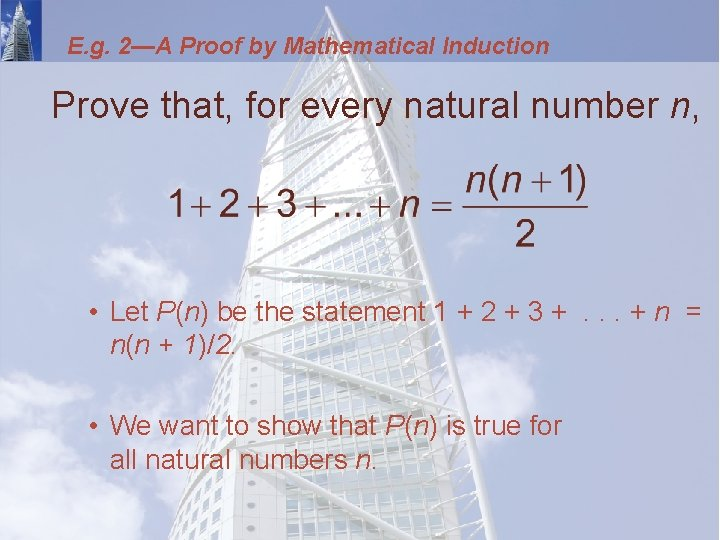E. g. 2—A Proof by Mathematical Induction Prove that, for every natural number n, • Let P(n) be the statement 1 + 2 + 3 +. . . + n = n(n + 1)/2. • We want to show that P(n) is true for all natural numbers n.E. g. 2—Proof by Mathematical Induction Step 1 We need to show that P(1) is true. • However, P(1) says that: • This statement is clearly true.E. g. 2—Proof by Mathematical Induction Step 2 Assume that P(k) is true. • Thus, our induction hypothesis is: • We want to use this to show that P(k + 1) is true. • That is,E. g. 2—Proof by Mathematical Induction Step 2 So, we start with the left side and use the induction hypothesis to obtain the right side: 1 + 2 + 3 +. . . + k + (k + 1) = [1 + 2 + 3 +. . . + k] + (k + 1)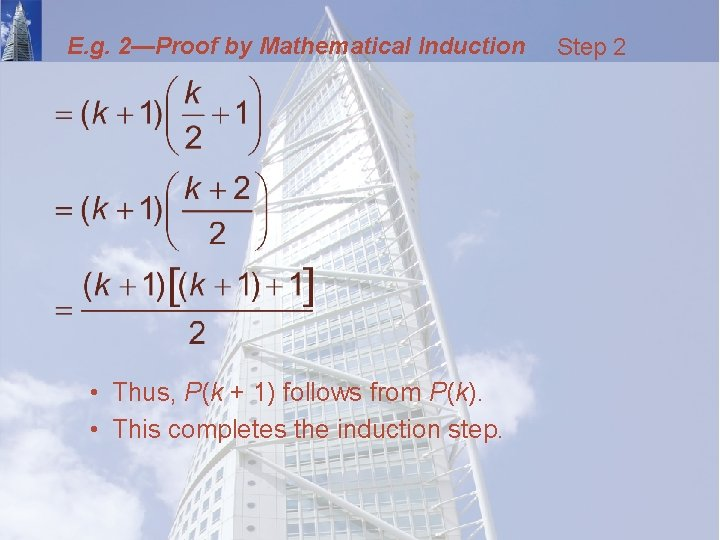E. g. 2—Proof by Mathematical Induction • Thus, P(k + 1) follows from P(k). • This completes the induction step. Step 2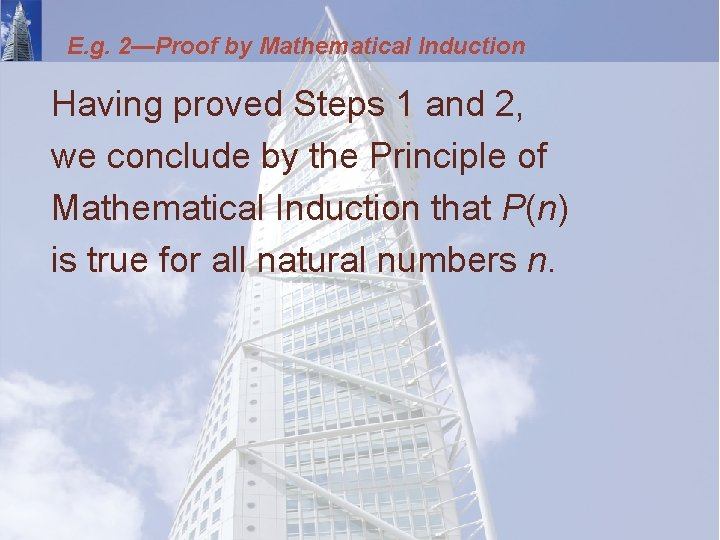E. g. 2—Proof by Mathematical Induction Having proved Steps 1 and 2, we conclude by the Principle of Mathematical Induction that P(n) is true for all natural numbers n.Sums of Powers Formulas for the sums of powers of the first n natural numbers are important in calculus. • Formula 1 is proved in Example 2. • The others are also proved using mathematical induction.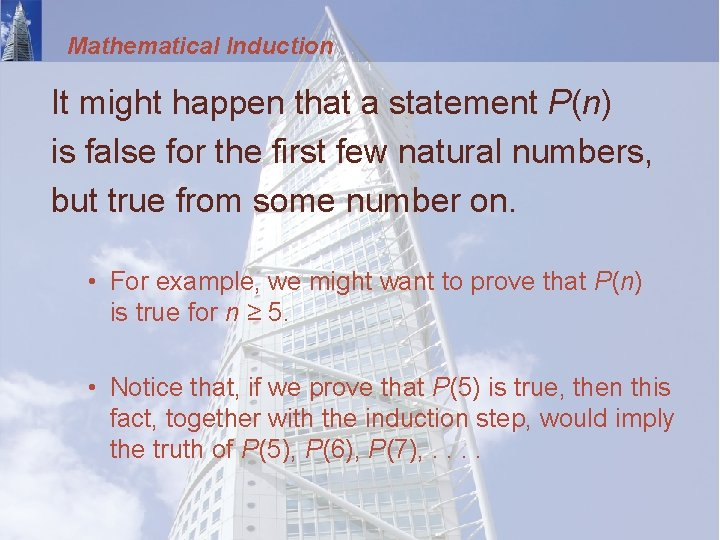Mathematical Induction It might happen that a statement P(n) is false for the first few natural numbers, but true from some number on. • For example, we might want to prove that P(n) is true for n ≥ 5. • Notice that, if we prove that P(5) is true, then this fact, together with the induction step, would imply the truth of P(5), P(6), P(7), . .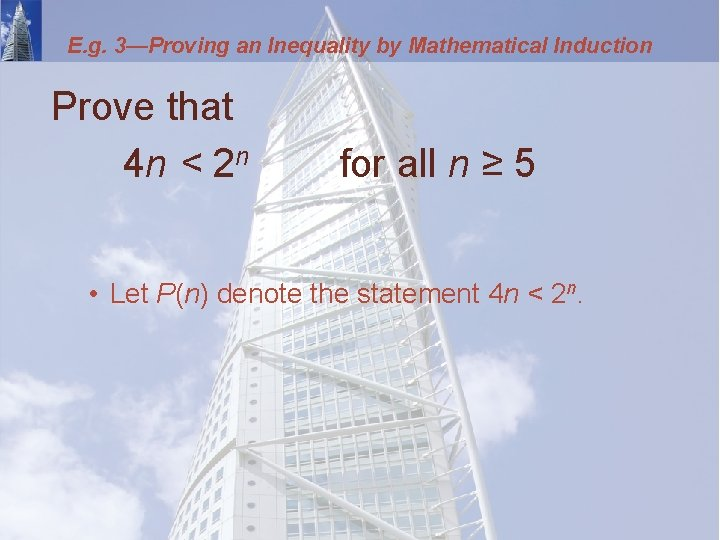E. g. 3—Proving an Inequality by Mathematical Induction Prove that 4 n < 2 n for all n ≥ 5 • Let P(n) denote the statement 4 n < 2 n.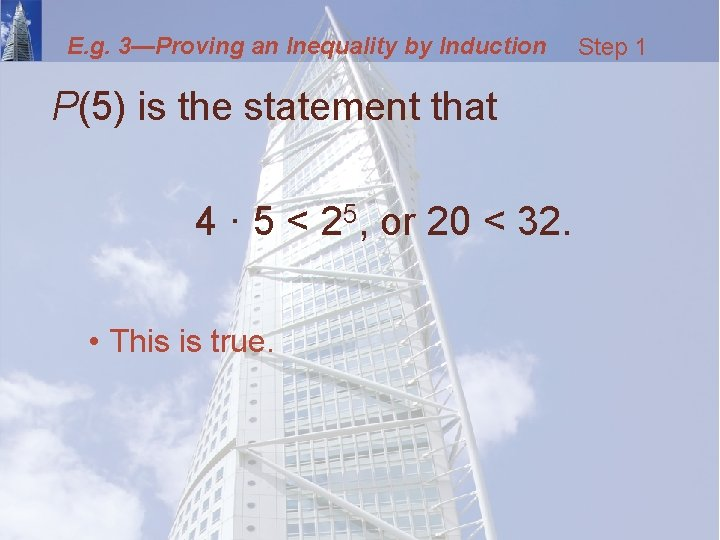E. g. 3—Proving an Inequality by Induction P(5) is the statement that 4 · 5 < 25, or 20 < 32. • This is true. Step 1E. g. 3—Proving an Inequality by Induction Step 2 Assume that P(k) is true. • Thus, our induction hypothesis is: 4 k < 2 k • We want to use this to show P(k + 1) is true. • That is, 4(k + 1) < 2 k + 1 • So, we start with the left side of the inequality and use the induction hypothesis to show that it is less than the right side.E. g. 3—Proving an Inequality by Induction Step 2 For k ≥ 5, we have: 4(k + 1) = 4 k + 4 (Distributive Property) < 2 k + 4 (Induction hypothesis) < 2 k + 4 k (Since 4 < 4 k)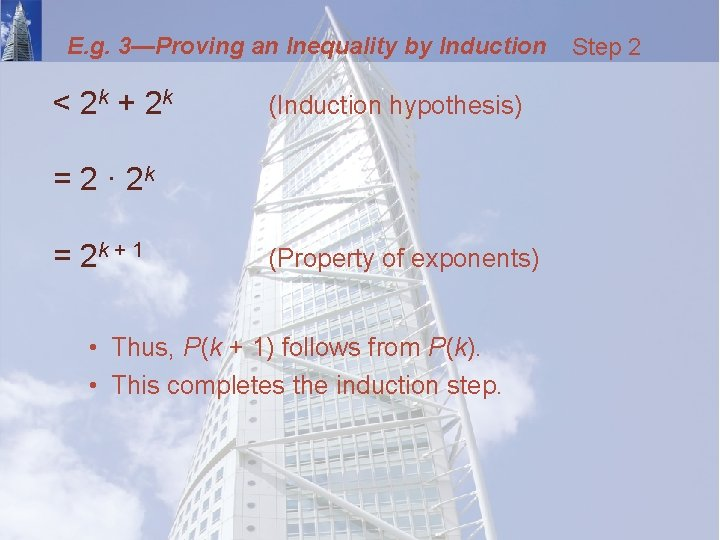E. g. 3—Proving an Inequality by Induction < 2 k + 2 k (Induction hypothesis) = 2 · 2 k = 2 k + 1 (Property of exponents) • Thus, P(k + 1) follows from P(k). • This completes the induction step. Step 2E. g. 3—Proving an Inequality by Induction Having proved Steps 1 and 2, we conclude by the Principle of Mathematical Induction that P(n) is true for all natural numbers n ≥ 5.